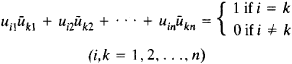Unitary Matrix

(redirected from Unitary matrices)
Also found in: Dictionary.

unitary matrix

[′yü·nə‚ter·ē ′mā·triks]
(mathematics)
A matrix whose inverse is equal to the complex conjugate of its transpose.

Unitary Matrix

A unitary matrix of order n is an n × n matrix [uik] with complex entries such that the product of [uik] and its conjugate transpose [ūki] is the identity matrix E. The elements of a unitary matrix satisfy the relationsThe unitary matrices of order n form a group under multiplication. A unitary matrix with real entries is an orthogonal matrix.

References in periodicals archive ?
2] are unitary matrices, and the diagonal matrices [MATHEMATICAL EXPRESSION NOT REPRODUCIBLE IN ASCII] have nonnegative diagonal entries satisfying [MATHEMATICAL EXPRESSION NOT REPRODUCIBLE IN ASCII].
ultra] is rank-structured and unitary matrices preserve rank.
This facsimile of the 1950 edition by Clarendon Press includes all the original material, covering matrices, algebras, groups, the Frobenius algebra, the symmetric group, immanants and S-functions, the S-functions of special series, the calculation of the characters of the symmetric group, group characters and the construction of groups, continuous matrix groups and invariant matrices, and groups of unitary matrices.
Killip and Nenciu  describe an ensemble of n x n random unitary matrices whose eigenvalues are distributed according to the Gibbs distribution for n particles of the Coulomb gas on the unit circle.
The CMV and unitary matrices in general are linked to orthogonal polynomials on the unit circle for which a rich variety of literature is available; early contributions and references can be found in [1-3].
which, for unitary matrices, links closely to CMV matrices .
where U and V are unitary matrices, and [SIGMA] is a diagonal matrix where the diagonal entries are real and nonnegative and satisfy [[sigma].
A singular value decomposition of a non-zero complex N x N matrix B is an expression of B as a product B = V[SIGMA]W*, where V and W are unitary matrices in [C.
A quantum computation applies a unitary matrix to a vector , and quantum (logic) circuits are a notational shorthand for factorizations of such unitary matrices into more basic ones, corresponding to gates.
Unitary matrices A are characterized by [parallel] Ax [parallel] = [parallel] x [parallel] for all x [member of] [H.

Site: Follow: Share:
Open / Close